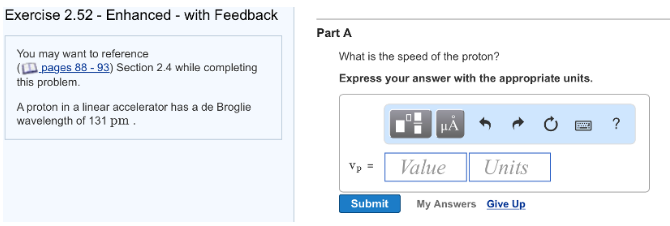Problem: A proton in a linear accelerator has a de Broglie wavelength of 131 pm. Part AWhat is the speed of the proton? Express your answer with the appropriate units.

FREE Expert Solution
84% (173 ratings)
FREE Expert Solution
84% (173 ratings)
Problem Details

A proton in a linear accelerator has a de Broglie wavelength of 131 pm.

Part A

What is the speed of the proton?What scientific concept do you need to know in order to solve this problem?

Our tutors have indicated that to solve this problem you will need to apply the De Broglie Wavelength concept. You can view video lessons to learn De Broglie Wavelength Or if you need more De Broglie Wavelength practice, you can also practice De Broglie Wavelength practice problems .

How long does this problem take to solve?

Our expert Chemistry tutor, Jules took 1 minute to solve this problem. You can follow their steps in the video explanation above.

What professor is this problem relevant for?

Based on our data, we think this problem is relevant for Professor Pierce's class at UA.

What textbook is this problem found in?

Our data indicates that this problem or a close variation was asked in . You can also practice practice problems .